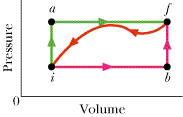# First Law of Thermodynamics (Eint = Q-W) question. I am only stuck on part (d)

zag4life
When a system is taken from state i to state f along path iaf in the figure, Q = 60 cal and W = 10 cal. Along path ibf, Q = 65 cal.(a) What is W along path ibf? (b) If W = -19 cal for the return path fi, what is Q for this path? (c) If Eint,i = 11 cal, what is Eint,f?(d) If Eint,b = 27 cal what is Q for path ib?(e) For the same value of Eint,b, what is Q for path bf?

## Homework Equations

Eint= internal energy of the system; Q= heat; W= work
I have used Eint= Q - W to solve most of the problem.

## The Attempt at a Solution

I only am marked wrong on part d which is very frustrating. So far I have:
(a)Wibf = 15 cal because W= Q-E (b) Qfi= -69 cal because Q= -(E)+W (c) Eint,f= 61 cal because Eint,f= Eint+Ei (d) Qib= ? (e) Qbf= 34 because Eint,f =E-Eint,b
Any thoughts?

Last edited:

Homework Helper
Gold Member
Hi, zag4life. Welcome to PF.

How does the work for i $\rightarrow$b compare to the work for i$\rightarrow$b$\rightarrow$f?

zag4life
Well, from b to f, because the volume does not change, work is equal to zero. The work for i,b,f would be the same as the work for i,b I think.

Homework Helper
Gold Member
Good. So, use that to get Q for ib.